# Quantifier

(diff) ← Older revision | Latest revision (diff) | Newer revision → (diff)
The general name for a logical operation that constructs from a predicate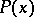a statement characterizing the domain of validity of. In mathematical logic, the most widely used quantifiers are the universal quantifier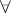and the existential quantifier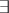. The statement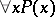means that the domain of validity ofis the same as the domain of values of the variable. The statement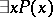means that the domain of validity ofis non-empty. If one is interested in the behaviour of the predicatenot on the whole domain of values of, but only on the part singled out by a predicate, then one often uses the restricted quantifiers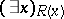and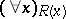. In this case, the statementmeans the same as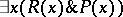, whilehas the same meaning as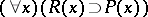, whereis the conjunction sign and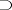is the implication sign.
More generally, the model-theoretic interpretation of an arbitrary "quantifier"(with the same syntactic behaviour asand) can (according to A. Mostowski) be given by a mapping associating with each modela class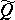of subsets of. Then one stipulates as a truth-definition forthat, e.g., a sentence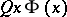holds inif and only if the set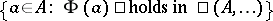is in. Thus, with the existential quantifieris associated the class of non-empty subsets ofand with the universal quantifieris associated the class. However, there are many more possible quantifiers, e.g. given by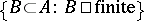,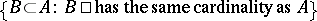(the Chang quantifier),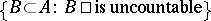, etc. This set-up can be generalized to "quantifiers" binding more than one variable occurring in more than one formula (example: the equi-cardinality quantifierbinding two variablesandin two formulas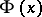and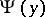, yielding the formula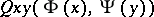, which is interpreted by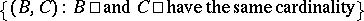). Even more general is the Lindström quantifier. And each quantifier has its own logic.